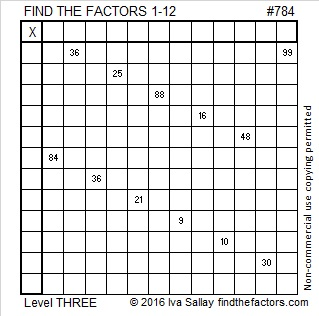# 784 and Level 3

If you know the multiplication facts up to 12 x 12, then it is obvious that 784 is divisible by 7. However, 784 is divisible by a whole lot more numbers than that. 784 can actually be evenly divided by 15 different numbers. Hmm, 15, that’s an odd number. A number’s factors always come in pairs. One of its factor pairs must contain the same factor twice, meaning 784 is a perfect square!

• 784 is a composite number.
• Prime factorization: 784 = 2 x 2 x 2 x 2 x 7 x 7, which can be written 784 = (2^4) x (7^2)
• The exponents in the prime factorization are 4 and 2. Adding one to each and multiplying we get (4 + 1)(2 + 1) = 5 x 3 = 15. Therefore 784 has exactly 15 factors.
• Factors of 784: 1, 2, 4, 7, 8, 14, 16, 28, 49, 56, 98, 112, 196, 392, 784
• Factor pairs: 784 = 1 x 784, 2 x 392, 4 x 196, 7 x 112, 8 x 98, 14 x 56, 16 x 49, or 28 x 28
• 784 is a perfect square. √784 = 28But that’s not the only thing remarkable about this perfect square: √784 is 28, the 7th triangular number, so like all other squared triangular numbers 784 has this additional property:Just as 784 is a perfect square, five of the twelve clues in today’s puzzle are also perfect squares. But don’t let that fact trick you into writing the same factor in both the first column and the top row every time!Print the puzzles or type the solution on this excel file: 12-factors-782-787

—————————————

Here’s more about the number 784:

784 can be written as the sum of three squares in exactly one way:

• 24² + 12² + 8² = 784

784 is also a palindrome in Bases 13, 17, and 27:

• 484 BASE 13; note that 4(169) + 8(13) + 4(1) = 784
• 2C2 BASE 17 (C is 12 base 10); note that 2(289) + 12(17) + 2(1) = 784
• 121 BASE 27; note that 1(27²) + 2(27) + 1(1) = 784

—————————————